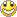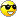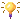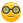Some new stuff
1. Arduino Park Control
2. Arduino USB2serial programming the bare bone
3. Arduino Projects Videos added
4. Arduino Xbee projects
5. Arduino dB Meter
6. Trying to get the IOIO going.
7. IOIO is going....bluetooth8. Attiny 2313 bluetooth bee RC car
9. Compass and temperature added
10. AVR programmer
11. USB2Serial and Ethernet POE
12. Arduino LPG Sensor
13. Program the Arduino Mini 05
14. Lipo Rider Pro in action
15. EZ Robot Builder kit arrived 10 Nov 2012
16. Arduino VA Meter!
17. 05 Nov 2012 Project Sentry Gun.
18. Attiny85 PIR Sensor 10 Dec 2012
19. 0-30VDC3A and 2-28VDC10A bench PSU's20. 19 Dec 2012 Arduino Voltmeter LCD
21. 29 Dec 2012 Arduino Tachometer&Speedometer LCD
22. 03 Jan 2013 Arduino AC Phase Control.
23. 18 Jan 2013 Arduino Distance Meter
24. 22 Jan 2013 Arduino Digital Clock and Date
25. 31 Jan 2013 Arduino Digital Clock and Date 8x2 LCD
26. Feb 2013 LCD and Backpack
27. Feb 2013 7 Segment Serial Clock Sparkfun. Distance Sensor with "newping" library
28. Feb 2013 Lelo Remote
29. 01/03/2013 Arduino Adafruit 7 Seg Digital Clock with RTC and backpack
30. Arduino Frequency Meter 26/03/2013
31. Another RC  Car controlled by Arduino 06/04/2013
32. My quadcopter project update 25 April 2013
33. My Aqua Quad Copter Flying 26 April 201334. Another monster RC truck;strong one! 11July201335. X-Frame Quad Copter 31 July 201336. Arduino GSM Sheild added.28/8/2013
37. 1.8inch TFT screen 13/09/201338. 04/11/2014 added PID Soldering Iron Control
39. Added bluetooth servo control 04/11/2014
40. ESP8266 first try.16/03/2015
41. Arduino Uno Quadcopter 19 May 2015
42. IOT WemosD1 Amp MeterArduino LM35 Temp Sens LCD 8by2.docx

Arduino LM35 Temp Sens LCD 8by2

Arduino LM35 Temp Sens LCD 8x2.

Nothing new, but I wanted to try the 8x2 LCD.Code:

#include //include Arduino LCD library

int temperature = 0;  //declare a variable to store obtained temperature

const char analog_pin = A0;   //assign analog_pin with analog pin A0

const char buzzer = 2;        //assign buzzer with digital pin 2

LiquidCrystal lcd(8,9,4,5,6,7);  //Initialize the LCD with:

//RS -->  digital pin 8

//E -->   digital pin 9

//DB4 --> digital pin 4

//DB5 --> digital pin 5

//DB6 --> digital pin 6

//DB7 --> digital pin 7

//RS, E, DB4, DB5, DB6 and DB7 are pins of LCD

//R/W to ground

//Setup

void setup()

{

pinMode(buzzer,OUTPUT);  //set buzzer (or pin 2) as output

pinMode(analog_pin,INPUT); //set analog_pin (or pin A0) as input

analogReference(INTERNAL); //configure the ADC reference voltage to 1.1V (internal built-in)

lcd.begin(8,2);  //configure for 2 x 8 LCD (2 rows, 8 columns) operation

lcd.setCursor(0,0);  //initialize the cursor at 1st position of the 1st line

lcd.print(" Temp(C)"); //print "TEMP(C)" on LCD starting from position of cursor

}

void loop()

{

temperature = get_temperature();  //get the temperature

if(temperature>300)  //test if the temperature > 32.5 celsius

beep();  //if yes, then activate the buzzer

lcd.setCursor(2,1);  //set the cursor at 1st position of the 2nd line

lcd.print(temperature/10,DEC);  //Starting from position of cursor, print the round number part of temperature as decimal on LCD

lcd.print('.');  //print a dot '.' on LCD

lcd.print(temperature%10,DEC); //print the decimal part of temperature as decimal on LCD

delay(300);  //short delay between acquisition of temperature

}

void beep()

{

//buzzer sound pattern

digitalWrite(buzzer,HIGH);  //buzzer on

delay(100);  //short delay

digitalWrite(buzzer,LOW);  //buzzer off

delay(100);  //short delay

}

//Get temperature subroutine

int get_temperature()

{

/*****get temperature subroutine*****

-The formula to convert ADC reading to temperature is shown below:

Temperature = 100 x ADC reading x 1.1 / 1024 x 10

-The temperature sensor (LM35DZ or LM35DT) sensing range is between 0 - 100 celsius

-Sensitivity --> 10mv per celsius

-With the sensing range --> 0 - 100 celsius, the LM35DZ/DT voltage output --> 0 - 1V

-With analog voltage reference = 1.1, maximum ADC value obtained = 1 / 1.1 * 1024 = 930. Hence, the ADC value range is between 0 - 930

-A multipler 1.1 have to be introduced so that we can get 100 celsius when the value is 930 as 930 x 1.1 --> approximately 1024

-Another multipler 10 also have to be introduced, so we can obtain the temperature in one decimal place instead of rounded number without decimal place

eg:

As temperature --> 61.5 celsius is sensed,

-if multiplier 10 is introduced, average temperature store in a variable average_temperature is 615 instead of 61

-the round number part is obtained using average_temperature / 10 --> 61

-the decimal number part is obtained using average_temperature % 10 --> 5

-By manipulating this, we can display the temperature with one decimal place

************************************/

float adc_value = 0;  //declare a variable to store ADC value

float total_temperature = 0;  //declare a variable to store sum of multiple temperatures

//for average temperature calculation

int average_temperature = 0;  //declare a variable to store the average temperature calculated

for(int count =0; count<100; count++)  //obtainning the sum of multiple temperatures (100 samples in this case)

{

total_temperature += adc_value/1024*100*1.1*10;  //accumulate the sum of temperatures untill all samples are taken, same as total_temperature = total_temperature + adc_value/1024*100*1.1*10;

}

average_temperature = int(total_temperature / 100);  //calculate the average temperature, convert it from float to integer

return average_temperature;  //return average temperature to the main

}

The following picture show own veroboard lm35 temp breakout board with my own 8x2 sheild:And then by means of copy and paste I learned something about void...() instruction.

So I changed the code again to make provision for ledpin blink if the temp is higher than a set value and will blink with the beep instruction.

Here the changed code again:

#include <LiquidCrystal.h> //include Arduino LCD library,NB not with backpack library.
int temperature = 0;       //declare a variable to store obtained temperature
const char analog_pin = A0;   //assign analog_pin with analog pin A0
const char buzzer = 2;        //assign buzzer with digital pin 2
const int ledPin = 13;
LiquidCrystal lcd(8,9,4,5,6,7);  //Initialize the LCD with:
//RS -->  digital pin 8
//E -->   digital pin 9
//DB4 --> digital pin 4
//DB5 --> digital pin 5
//DB6 --> digital pin 6
//DB7 --> digital pin 7
//RS, E, DB4, DB5, DB6 and DB7 are pins of LCD
//R/W to ground
//Setup
void setup()
{
pinMode(ledPin,OUTPUT);//set ledPin13 as output
pinMode(buzzer,OUTPUT);  //set buzzer (or pin 2) as output
pinMode(analog_pin,INPUT); //set analog_pin (or pin A0) as input
analogReference(INTERNAL); //configure the ADC reference voltage to 1.1V (internal built-in)
lcd.begin(8,2);  //configure for 2 x 8 LCD (2 rows, 8 columns) operation
lcd.setCursor(0,0);  //initialize the cursor at 1st position of the 1st line
lcd.print("  Temp"); //print "TEMP(C)" on LCD starting from position of cursor
}
void loop()
{
temperature = get_temperature();  //get the temperature
if(temperature>312)  //test if the temperature > 31.2 celsius
beep();  //if yes, then activate the buzzer
if(temperature>312)  //test if the temperature > 31.2 celsius
blink_LED();//if yes, activate ledPin
lcd.setCursor(2,1);  //set the cursor at 1st position of the 2nd line
lcd.print(temperature/10,DEC);  //Starting from position of cursor, print the round number part of temperature as decimal on LCD
lcd.print('.');  //print a dot '.' on LCD
lcd.print(temperature%10,DEC); //print the decimal part of temperature as decimal on LCD
lcd.print((char)223);     // Output the degrees character
lcd.print("C");
delay(300);  //short delay between acquisition of temperature
}
void beep()
{
//buzzer sound pattern
digitalWrite(buzzer,HIGH);  //buzzer on
delay(50);  //short delay
digitalWrite(buzzer,LOW);  //buzzer off
delay(50);  //short delay
}
{
digitalWrite(ledPin,HIGH);  //led on
delay(50);  //short delay
digitalWrite(ledPin,LOW);  //led off
delay(50);  //short delay
}
//Get temperature subroutine
int get_temperature()
{
/*****get temperature subroutine*****
-The formula to convert ADC reading to temperature is shown below:
Temperature = 100 x ADC reading x 1.1 / 1024 x 10
-The temperature sensor (LM35DZ or LM35DT) sensing range is between 0 - 100 celsius
-Sensitivity --> 10mv per celsius
-With the sensing range --> 0 - 100 celsius, the LM35DZ/DT voltage output --> 0 - 1V
-With analog voltage reference = 1.1, maximum ADC value obtained = 1 / 1.1 * 1024 = 930. Hence, the ADC value range is between 0 - 930
-A multipler 1.1 have to be introduced so that we can get 100 celsius when the value is 930 as 930 x 1.1 --> approximately 1024
-Another multipler 10 also have to be introduced, so we can obtain the temperature in one decimal place instead of rounded number without decimal place
eg:
As temperature --> 61.5 celsius is sensed,
-if multiplier 10 is introduced, average temperature store in a variable average_temperature is 615 instead of 61
-the round number part is obtained using average_temperature / 10 --> 61
-the decimal number part is obtained using average_temperature % 10 --> 5
-By manipulating this, we can display the temperature with one decimal place
************************************/

float adc_value = 0;  //declare a variable to store ADC value
float total_temperature = 0;  //declare a variable to store sum of multiple temperatures
//for average temperature calculation
int average_temperature = 0;  //declare a variable to store the average temperature calculated

for(int count =0; count<100; count++)  //obtainning the sum of multiple temperatures (100 samples in this case)
{